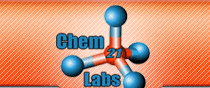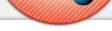Tutorials Textbooks are filled with guides, flow-charts, maps, approaches, patterns, pathways, etc. that build and connect knowledge and facts in complex ways. It is this scaffolding of 'complex thinking' that allows the learner to engage in critical thinking that ultimately results in correct answers to novel questions. Unfortunately, many learners fail to accumulate this information at the appropriate time in the learning process. Assignable online exercises that require students to actively use these learning pathways have been developed by Chem21Labs. General Chemistry Arguably a difficult, but very important, concept to master in the first semester General Chemistry course is the Factor-Unit Method (sometimes referred to as Dimensional Analysis). This method of solving problems by cancelling units is presented in four to five chapters in most textbooks as follows: English and Metric units of mass, length, area (length2) and volume are presented along with their conversion factors. Cubic length (length3) is presented and its conversion factor to volume is given as 1 mL = 1 cm3. In addition, density is introduced as a conversion factor between volume and mass. Moles and molecules are presented along with Avogadro's number. Also, the conversion factor of molar mass (derived from the Periodic Table) is introduced to allow the conversion between grams and moles. Students are taught how to balance equations and coefficient ratios are used in Factor-Unit problems as conversion factors between moles. At this point, a student is expected to solve a complex problem like If 3.45 ft3 of Hydrogen gas is burned in excess Oxygen, what volume (in nL) of water can be produced once the water vapor condenses to produce a liquid? Density of water = 1.00 g/mL Students are introduced to Molarity as the conversion factor between moles of a pure substance and Liters of a solution (usually aqueous) where the pure substance is the solute. At this point, a student is expected to solve a complex problem like If 34.5 mL of a 0.987 M NaCl is added to 30.2 mL of a 0.888 M AgNO3, how many mg of AgCl will be produced? Some students experience difficulty with the more complex questions like the ones above because they have not constructed a "personal" concept map from information presented over several weeks and chapters. These particular students benefit from pre-constructed HTML5-JS concept maps that are interactive and assignable. This "Learning Pathway" consists of clickable units that the student selects to "set up" a Dimensional Analysis problem. Next, the student must place the correct numbers in front of the units and label the units. The Map is also used to "set up" problems whose answers require two units, like density, molar mass, molar ratios or Molarity. It is important to note that "Learning Pathways" require students to answer a Dimensional Analysis problem exactly as they would find it answered in a text book, answer guide or from their instructor. By forcing students to use a method that is "universally" taught in textbooks and classrooms, the learner is supplied with a guaranteed path to success that reinforces the presentation strategies used in most textbooks and classrooms. Click here to view Chem21Labs' Interactive Unit Maps. A second important concept in General Chemistry I is calculating the limiting reagent in a reaction and then determining the theoretical yield and/or amount of an excess reagent. An interactive TRQ (iTRQ - Limiting Reagent) has been created where students can "practice until they are successful." Either they correctly answer the posed question in a certain amount of time, or they correctly work through a guided solution 5 times. In General Chemsitry II, the calculation of pH is probably the most difficult to master. The Interactive pH Calculator "guides" students through this challenging maze by first having the student select the specie(s) impacting pH: Strong Acid Strong Base Weak Acid Weak Base Strong Acid + Strong Base Strong Acid + Weak Base Strong Acid + Weak Acid Strong Base + Weak Acid Strong Base + Weak Base Weak Acid + Weak Base Buffer Solution + Strong Acid Buffer Solution + Strong Base Then as needed, ICE tables are filled in by the students and their calculations are checked at every stage. Students can opt to neglect "x", but if the test fails they will have to use the quadratic equation. Finally, students must calculate the pH, pOH, [H3O+] and [OH-]. Phase diagrams and heating curves couples the phase changes in a phase diagram with the calculations used in a Heating Curve problem . . . . 64.5 g of ice at -25°C is heated to water at 89.9°C. How much energy in Joules was added? Interconversion of Concentration Units provides an interactive tutorials where students discover the relationship between solute, solvent and solution. Additional "connections" (mass solute, moles solute, kg solvent, moles solvent, Liters solution) provide drag/drop components that are manipulated to create the various concentration terms: Molarity, molality, mass percent, ppm, and mole fraction. Finally, students must solve a problem like . . . . 12.5 g of NaCl is dissolved in 20.0 g water. Calculate the molality of the resulting solution if the density is 1.12 g/mL. Organic Chemistry Many students struggle when it comes to drawing organic mechanisms . . . . Chem21Labs has created re-assignable, interactive animations that, over time, will build the ability to write mechanisms for a variety of reactions. Once mastered, this same ability can be applied to any reactant containing the required functional group. Click Writing Organic Mechanisms to draw the curved arrows in the following reactions: H2O + HCl Acetone + HBr Cyclohexene + Br2 A stereochemistry tutorial contains chiral molecules that can be rotated in 3D so students can determine R/S configuration or whether two stereoisomers are enantiomers or diastereomers. Tutorials and iTRQs use 21st Century technology to create a "forced engagement" at a relatively high level of cognitive thought. Teacher can use this "coach-like" approach to schedule the intensity, duration and repetition schedule of academic work. Just like an athlete, some students may not enjoy the process . . . . but, all will be thrilled with the results.